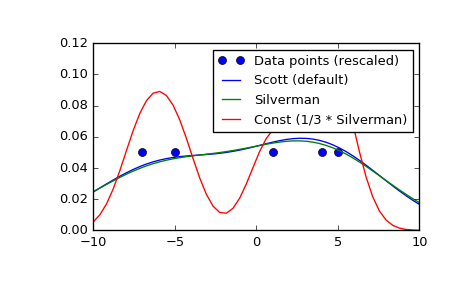# scipy.stats.gaussian_kde.set_bandwidth¶

gaussian_kde.set_bandwidth(bw_method=None)[source]

Compute the estimator bandwidth with given method.

The new bandwidth calculated after a call to set_bandwidth is used for subsequent evaluations of the estimated density.

Parameters: bw_method : str, scalar or callable, optional The method used to calculate the estimator bandwidth. This can be ‘scott’, ‘silverman’, a scalar constant or a callable. If a scalar, this will be used directly as kde.factor. If a callable, it should take a gaussian_kde instance as only parameter and return a scalar. If None (default), nothing happens; the current kde.covariance_factor method is kept.

Notes

New in version 0.11.

Examples

>>> import scipy.stats as stats
>>> x1 = np.array([-7, -5, 1, 4, 5.])
>>> kde = stats.gaussian_kde(x1)
>>> xs = np.linspace(-10, 10, num=50)
>>> y1 = kde(xs)
>>> kde.set_bandwidth(bw_method='silverman')
>>> y2 = kde(xs)
>>> kde.set_bandwidth(bw_method=kde.factor / 3.)
>>> y3 = kde(xs)

>>> import matplotlib.pyplot as plt
>>> fig, ax = plt.subplots()
>>> ax.plot(x1, np.ones(x1.shape) / (4. * x1.size), 'bo',
...         label='Data points (rescaled)')
>>> ax.plot(xs, y1, label='Scott (default)')
>>> ax.plot(xs, y2, label='Silverman')
>>> ax.plot(xs, y3, label='Const (1/3 * Silverman)')
>>> ax.legend()
>>> plt.show()#### Previous topic

scipy.stats.gaussian_kde.resample

#### Next topic

scipy.stats.gaussian_kde.covariance_factor